## 1 Introduction

In his well-known book Thought Experiments (1992), R.A. Sorensen provides two modal-logical schemata for two different types of ‘destructive’ thought experiments, baptised the Necessity Refuter and the Possibility Refuter. Regarding his schemata, Sorensen (1992, p. 132) advances the following caveat:

Don’t worry about whether this is the uniquely correct scheme. The adequacy of a classification system is more a question of efficiency and suggestiveness. A good scheme consolidates knowledge in a way that minimizes the demand on your memory and expedites the acquisition of new knowledge by raising helpful leading questions.

Both the Necessity Refuter and the Possibility Refuter consist of five premises, which are claimed to be inconsistent (ibid,, p. 135, 153). Besides clarity about the logical structure of thought experiments, another virtue of the modal-logical schemata is, Sorensen submits, the following (ibid, p. 136):

Since the above five premises are jointly inconsistent, one cannot hold all five. This means that there are at most five consistent responses to the set.

Sorensen then discusses the five possible responses, each of which rejects one premise.

We concur with Sorensen that systematisations of the arguments accompanying thought experiments should be judged by their usefulness, such as classifying different responses. If the premises are inconsistent, then at least one premise must be given up; but if they are consistent, they can all be held. Indeed, we claim that sticto sensu both these modal-logical schemata are consistent, undermining the usefulness of the systematisation.

We shall consider first Sorensen’s Necessity Refuter (Sect. 2), and then his Possibility Refuter (Sect. 3). We then move to Häggqvist (2009) different modal-logical schema of a destructive thought experiment, which we also show to be invalid by proving that stricto sensu it also is consistent. (Sect. 4). We conclude by indicating the bearing of our analyses on responses to thought experiments, in particular whether new avenues to respond have became available (Sect. 5).

## 2 Sorensen’s Necessity Refuter

According to Sorensen (1992, p. 153), Necessity Refuters aim to demonstrate that “the source is too closed-minded, that it rules out genuine possibilities”. Sorensen uses the phrase ‘source’ (S below) as the source of modal propositions, such as some theory, hypothesis or principle, which justifies or is committed to certain modal propositions. These thought experiments are dubbed ‘destructive’ because they are designed to undermine that source.

### 2.1 Formalisation

The following five premises are supposed to capture the logical structure of the Necessity Refuter (ibid., Sorensen’s terminology):(2.1)

Proposition C describes the possible counterfactual situation imagined in the thought experiment (5. Content Possibility, 3. Counterfactual);is some necessity of interest implied by S (2. Necessity Extractor); and W is what ensues in situation C according to S via its implication I (3. Counterfactual). But W is intuitively ruled out as impossible (4. Absurdity). Hence we are invited to reject S (1. Modal Source Statement), which is then considered to be refuted with the aid of the thought experiment.

For illustration, consider Searle’s Chinese Room Argument: the destructive thought experiment supposed to refute the thesis of strong artificial intelligence, which claims that an algorithm passing the Turing Test (by displaying linguistic behaviour functionally indistinguishable from a native speaker) also understands that language. The narrative is that Searle is confined to a room together with only a database of Chinese symbols, and a rulebook that lists how to correlate Chinese symbols with other Chinese symbols. From outside of the room, people can put in Chinese symbols (imagine them written down on paper), which symbols Searle then looks up in the rulebook, mapping them to other symbols, which are then put out again. The input consists (unbeknownst to Searle) of questions and the output of appropriate answers to these questions: the Chinese Room with Searle in it passes the Turing Test. Searle claims that if he were to perform this task of manipulating these symbols, which are incomprehensible and meaningless for him, he would remain a Chinese illiterate, someone who does not understand Chinese.

Damper (2006) has applied Sorensen’s schema to Searle’s Chinese Room thought experiment (without noting our point below, about the schema being incorrect). S is the hypothesis of strong artificial intelligence; I is taken to be the implementation of the Chinese algorithm; C is Searle hand-implementing the algorithm and behaving indistinguishably from a native Chinese language user; and W is Searle understanding Chinese. Schema (2.1), then, straightforwardly reproduces the above narrative. Searle solves the inconsistency by rejecting S, whereas those disagreeing with Searle can choose to reject one or more of premises 2–5 of (2.1), and their responses are then classified accordingly.

Let us return to the modal-logical schema. The modal operators(necessity) and(possibility) are the usual alethic ones; they are inter-definable via the standard equivalence of Aristotelian origin: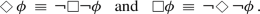(2.2)

The notation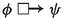, also known as the ‘box-arrow’, is spot-on in light of the notation of the strict implication (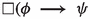)), and was introduced (and given a thorough analysis) by Lewis (1973) to capture subjunctive conditionals: if $$\phi$$ were the case, then $$\psi$$ would be the case. Since we often use them with a false antecedent (‘contrary to fact’), they are also called counter-factuals.

Sorensen leaves things here and moves on to applying this schema, thus without providing neither proof of the claimed inconsistency of (2.1) nor entering into the semantics of (2.1). We shall consider precisely these issues now.

To obtain truth-conditions for counterfactuals, Lewis adopts a comparative similarity relation: world w is more similar or at least as similar to the actual world $$w_{@}$$ than $$w'$$ is to $$w_{@}$$: $$\,w\preccurlyeq _{@}w'$$. This similarity judgement relies on what is relevant to the antecedent $$\phi$$ of the counterfactual. Therefore it would be better to speak of $$\phi$$-similarity and to write: $$\;w\preccurlyeq _{@,\phi }w'\;$$. When this relation meets the six conditions that Lewis (1973, p. 48) imposes, we can, for every proposition $$\phi$$, subdivide the set of all accessible worlds $$\mathcal {W}^{@}$$ into a finite set of n regions of worlds: first a region with worlds most $$\phi$$-similar to $$w_{@}$$, which we shall denote as $$\mathcal {S}^{0}_{\phi }$$ (alluding to Similar); then regions of worlds less and less $$\phi$$-similar to $$w_{@}$$ (or equally dissimilar), leading to disjoint sets $$\mathcal {S}^{j}_{\phi }$$ ($$j=0,1,\ldots ,n$$), ending with the region $$\mathcal {S}^{n}_{\phi }$$ of worlds most $$\phi$$-dissimilar to $$w_{@}$$. The sets inaccessible from $$w_{@}$$ are considered to be too dissimilar from $$w_{@}$$ to merit consideration in counterfactuals. Let us call $$\mathcal {S}_{\phi }$$ the union of all the disjoint sets $$\mathcal {S}^{j}_{\phi }$$, i.e. $$\mathcal {S}_{\phi }=\cup \mathcal {S}^{j}_{\phi }$$. The set $$\mathcal {W}^{@}$$ is not exhaustively subdivided by the regions: the complement $$\mathcal {W}^{@} \!\setminus \mathcal {S}_{\phi }$$ of accessible but $$\phi$$-dissimilar worlds is vast.

We follow Lewis by assuming that the union-set of $$\phi$$-similarity spheres $$\mathcal {S}_{\phi }$$ is normal, which is to say that it is not empty; we do not impose any other constraints from Lewis’ list (1973, p. 120). (Of course we follow Lewis (1973, p. 14) by imposing on $$\mathcal {S}_{\phi }$$ that it is centred around $$w_{@}$$, nested, and closed under the formation of unions and intersections.) Specifically, $$\mathcal {S}_{\phi }$$ is not totally reflexive, as Lewis (ibid.) calls it. This would entail that $$\mathcal {S}_{\phi }$$ coincides with $$\mathcal {W}^{@}$$, or even with $$\;\mathcal {W}\supseteq \mathcal {W}^{@}$$. Rather the contrary! There are lots and lots of accessible worlds that we judge to be too dissimilar from $$w_{@}$$ in the light of what is relevant for $$\phi$$ to permit them membership in any region $$\mathcal {S}^{j}_{\phi }$$. Which is to say that $$\mathcal {W}^{@}\!\setminus \!\mathcal {S}_{\phi }$$ is vast and therefore far from empty. We call them $$\phi$$-irrelevant worlds.

We also follow Lewis (1973, p. 16) by taking counterfactualto be true in the actual world $$w_{@}$$ iff (i) there are no accessible $$\phi$$-similar $$\phi$$-worlds (vacuous truth), or (ii) there are accessible $$\phi$$-similar $$\phi$$-worlds in some $$\phi$$-similarity set, $$\mathcal {S}_{\phi }^{k}$$ say, and in every world in that set, the material conditional $$\;\phi \varvec{\,\longrightarrow \,}\psi \;$$ is true — since $$\phi$$ is there true, so is $$\psi$$: $$\,(\mathcal {W}^{@}\cap \mathcal {S}_{\phi }^{k})\subset \mathcal {W}_{\psi }$$. In terms of sets of possible worlds:

\begin{aligned} \begin{array}{ll} {}\text {(i)}&{} \mathcal {S}_{\phi }\cap \mathcal {W}^{@}_{\phi } =\varnothing ,~~\text {or}\\ {}\text {(ii)}&{} \varvec{\exists }\,k\in \{0,1,\ldots ,n\}: \varnothing \subset \mathcal {S}^{k}_{\phi }\quad \text {and}\quad (\mathcal {S}^{k}_{\phi }\cap \mathcal {W}^{@}_{\phi })\cap \mathcal {W}^{@}_{\lnot \psi }=\varnothing \,. \end{array} \end{aligned}
(2.3)

Lewis (ibid.) points out two different cases of vacuous truth (i) [replacing Lewis’ world $$w_{i}$$ with our $$w_{@}$$, and his $$\cup \,\_{i}$$ with our $$\mathcal {S}_{\phi }$$], namely

Alternative (i) gives the vacuous case: either $$\phi$$ is true at no world, or it is true only at worlds outside $$\mathcal {S}_{\phi }$$. Then our counterfactual is vacuously true at $$w_{@}$$. We shall say in this case that $$\phi$$ is not entertainable, at $$w_{@}$$, as a counterfactual supposition.

Thus either (i.a) there is no accessible $$\phi$$-world, in which case $$\,\mathcal {W}^{@}_{\phi }=\varnothing$$ (see Fig. 1), or (i.b) there are accessible $$\phi$$-worlds, $$\,v\in \mathcal {W}^{@}_{\phi }\,$$ say, but they lie outside the ‘system of spheres’ surrounding $$w_{@}$$: $$\,v\not \in \mathcal {S}_{\phi }$$ (see Fig. 2). Both cases, (i.a) and (i.b), imply (i): $$\,\mathcal {S}_{\phi }\cap \mathcal {W}^{@}_{\phi }=\varnothing$$. Perhaps to belabour the obvious: since we have rejected that $$\mathcal {S}_{\phi }$$ is totally reflexive (see above), this keeps the complement $$\mathcal {W}^{@}_{\phi }\!\setminus \!\mathcal {S}_{\phi }$$ crowded with worlds (half the yellow band in all Figures, proposition C subsituted for $$\phi$$), and consequently the two conditions (i.a) and (i.b) for vacuous truth remain wide open. Again Lewis (1973, pp. 14–15) [again replacing his $$\cup \_{i}$$ with our $$\mathcal {S}^{j}_{\phi }$$]:

More important, I have left it open whether or not the set of all possible worlds is to be one of the spheres around each world j; or in other words, whether or not the union of $$\mathcal {S}_{\phi }$$ of all spheres around i is to exhaust the set of worlds; or, in still other words, whether or not every possible world is to lie with some or other sphere around j. If $$\mathcal {S}_{\phi }$$ is the set of all worlds, for each j, I will call $${\mathcal {S}_{\phi }}$$ universal.

Lewis leaves it open, so do we: our set of all and only $$\phi$$-similar worlds, $$\,\mathcal {S}_{\phi }=\cup _{k}\,\mathcal {S}_{\phi }^{k}\,$$, will never be universal (unless $$\phi$$ is a tautology, which in this paper, and in all thought experiments, it never is).

For our set of all worlds ($$\mathcal {W}$$), we take the set of all logically possible worlds—tweaked versions of our analyses can easily be provided if one takes $$\mathcal {W}$$ to be more restrictive, such as as the set of all metaphysically, or all nomologically possible worlds, as is nearly always the case for scientific thought experiments. To rehearse, $$\mathcal {W}^{@}\subseteq \mathcal {W}$$ is the set of all worlds accessible from the actual world; $$w_{@}$$ is accessible to itself ($$w_{@}\in \mathcal {W}^{@}$$); $$\phi$$ is possible iff there is some $$\phi$$-world accessible from $$w_{@}$$ (i.e. a member of $$\mathcal {W}^{@}$$) where $$\phi$$ is true ($$\varnothing \subset \,\mathcal {W}^{@}\cap \mathcal {W}_{\phi }$$); $$\mathcal {S}_{\phi }$$ is a set of worlds centered around $$w_{@}$$ that are similar to $$w_{@}$$ judged in the light of $$\phi$$; and $$\mathcal {W}^@\!\setminus \!\mathcal {S}_{\phi }$$ is the set of accessible worlds too dissimilar from $$w_{@}$$ for what is relevant for $$\phi$$ (these worlds are by definition $$\phi$$-irrelevant).

### 2.2 Consistency

We shall now show semantically that premises 1–5 of (2.1) are consistent by presenting a situation in which all five premises are true. But first, for the sake of understanding the situation better, we shall attempt to prove an inconsistency, as Sorensen may have envisioned.Footnote 1

Attempted Proof of Inconsistency. From 1 and 2 of (2.1), we obtainby modus ponens. Combinewith 5 (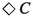) to obtain. To prove an inconsistency, one would like to proceed from here that the antecedent of 3 (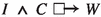) is satisfied, such that it follows that, which would contradict 4 (). However, in this last step, one has surreptitiously assumed the counterfactual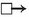to be substantively true, forgetting it can also be vacuously true. The attempted proof fails. We shall now proceed to show the consistency explicitly.

Using the fact that the material conditional $$\,\phi \varvec{\,\longrightarrow \,}\psi \,$$ is logically equivalent to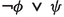, the standard truth-conditions of Sorensen’s five premises are as follows:(2.4)

Premise 1 of (2.1) is some claim (S) we assume to be true in the actual world. Note that since premise 2 states that some necessity () is extracted from S, S itself will contain modalities, and hence will have some truth-condition in terms of possible words. Since in the modal-logical reconstruction of the deduction accompanying the type of thought experiment under consideration, the issue howis extracted from S (to obtain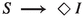) is irrelevant — we gloss over it.

We now proceed with the deduction of the consistency. The first disjunct of (tc2) contradicts (tc1), hence the second disjunct of (tc2) must hold: I is true in all worlds accessible from $$w_{@}$$: $$\mathcal {W}^{@}=\mathcal {W}^{@}_{I}$$. Then also $$\;\mathcal {S}^{k}_{I\wedge C}=\mathcal {S}^{@}_{C}\;$$ and $$\;\mathcal {W}^{k}_{I\wedge C}=\mathcal {W}^{@}_{C}\,$$. The truth-condition (tc3) of the counterfactual becomes $$\;(\mathcal {S}_{C}\cap \mathcal {W}^{@}_{C}) =\varnothing \;$$ or $$\;(\mathcal {S}^{k}_{C}\cap \mathcal {W}^{@}_{C})\cap \mathcal {W}^{@}_{\lnot W} =\varnothing$$. This is the truth-condition of the counterfactual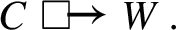(2.5)

The fulfilment of the generic second disjunct of (tc3) is depicted in Fig. 1 (having chosen $$n=1$$ for simplicity, carving $$\mathcal {S}_{C}$$ up in two sets: $$\mathcal {S}^{0}$$ in red shades, $$\mathcal {S}^{1}$$ in blue shades). We now consider the situation in which $$\;\mathcal {W}^{@}_{C}\cap \mathcal {S}_{C}=\varnothing$$: there is no C-world accessible from $$w_{@}$$ and similar to $$w_{@}$$. There are worlds accessible from $$w_{@}$$ where C is true, as (tc5) requires, but these are dissimilar to $$w_{@}$$: $$\,\varnothing \subset \mathcal {W}^{@}_{C}$$. This makes the counterfactual (2.5) vacuously true because the first disjunct of its truth-condition has been met. The situation is depicted in Fig. 2.

Truth-condition (tc4) requires that W is false in all worlds accessible from $$w_{@}$$: $$\mathcal {W}^{@}_{W}=\varnothing$$. Figure 3 depicts the fulfilment of the first disjunct of (tc3), and (tc4) together. The final truth-condition (tc5) requires there to be some world, call it v, accessible from $$w_{@}$$ where C is true: $$\;v\in \mathcal {W}^{@}_{C}\,$$. Since $$\mathcal {S}_{C}$$ does not contain C-worlds, world v must be dissimilar from $$w_{@}$$, so that $$\,v\not \in S_{C}\,$$ and $$\,v\in \mathcal {W}^{@}\!\setminus \!S_{C}$$. Nothing can prevent us from including C-worlds in $$\;\mathcal {W}^{@}\!\setminus \!\mathcal {S}_{C}\,$$, which is the upper yellow band in Fig. 3.

Since every premise of Sorensen’s pentad is true, this pentad is consistent. To make the pentad inconsistent, we must change it, which is what we shall proceed to do now.

### 2.3 Inconsistency

The standard counterfactual has a disjunctive truth-condition, of which the first makes the counterfactual vacuously true. This is what we have exploited in showing that Sorensen’s pentad is consistent. To obtain inconsistency, we add two premises:(2.6)

Let us justify 6 and 7 semantically. The truth-condition of 6 is such that if the truth-condition of the antecedent,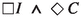, is fulfilled, then so must be the one of the consequent,. The truth-condition of the consequent is fulfilled if we can find some world in $$\mathcal {W}^{@}$$ where $$I\varvec{\,\wedge \,}C$$ is true. The second conjunct of the antecedent yields a world where C is true, say $$u\in \mathcal {W}^{@}$$. Since according to the first conjunct of the antecedent, I is true in every world in $$\mathcal {W}^{@}$$, it is also true in u. Then in world u both I and C are true, which means the conjunction $$I\varvec{\,\wedge \,}C$$ is also true in u. Hence premise 6 is a logical truth (in S1 and every stronger system).

Next, we consider premise 7 of (2.6). This is an instance of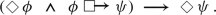(2.7)

Again, the consequent of the truth-condition of 7 is fulfilled if we can find a world in $$\mathcal {W}^{@}$$ where $$\psi$$ is true. Assuming that the antecedent of the truth-condition of 7 is fulfilled yields a world $$u\in \mathcal {W}^{@}$$ where $$\phi$$ is true, andis true in $$w_{@}$$. World u may be dissimilar to $$w_{@}$$, andmay be vacuously true in $$w_{@}$$, when $$\;\mathcal {S}_{\phi }\cap \mathcal {W}^{@}_{\phi }=\varnothing$$. We cannot derive that there is some accessible $$\psi$$-world, because $$\mathcal {W}_{\psi }$$ may be empty too, and again we have the situation depicted in Fig. 3. Hence unlike premise 6 of (2.6), premise 7 is not logically true because (2.7) is not one either.

If the second disjunct (ii) of the truth-condition ofis fulfilled, and we have some non-empty set of accessible worlds $$\mathcal {S}^{k}_{\phi }$$ that contains $$\phi$$-worlds, and these $$\phi$$-worlds are all $$\psi$$-worlds, then there are accessible $$\psi$$-worlds too, which makes the consequent of (2.6) true. Again everything hinges on whether the counterfactual is vacuously or substantively true.

The addition of premises (2.6) amounts to a single addition of only one premise, namely 7, since premise 6 has turned out to be logically true (that is, true in all interpretations of a given semantics, in this case S2 and stronger modal-logic systems). With this addition, we do obtain an inconsistent sextet, as we prove next.

Proof the Inconsistency of 1–7. From premises 1 and 2 of (2.1), we obtain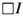by modus ponens. Combinewith premise 5 () to obtain the antecedent of premise 6, and then, again by modus ponens, we obtain. From the conjunction with premise 3, we obtain the antecedent of premise 7, and then, again by modus ponens, we deduce, which contradicts premise 4 (). Q.e.d.

## 3 Sorensen’s Possibility Refuter

Since we have treated Sorensen’s Necessesity Refuter nearly (if not entirely) to the point of tedium, and the diagnosis of his Possibility Refuter and Hägqqvist’s Counterfactual Refuter is essentially the same as with the Necessity Refuter, we shall proceed rather quickly.

The Possibility Refuter is the second type of destructive thought experiment that Sorensen presents. This Refuter aims to demonstrate that “the source is too open-minded, that it saddles us with spurious possibilities” (1992, p. 135). The logical schema is as follows (ibid., p. 153):(3.1)

In comparison to the Necessity Refuter, its premise 2 is replaced with $$2'$$, and its premise 5 () is replaced with $$5'$$. Sorensen’s claim is again that these five premises are inconsistent but he provides no proof for this claim.

A similar arrangement to show the consistency of Sorensen’s pentad (2.1) will also do to show the consistency of pentad (3.1). The truth-conditions of pentad (3.1) are as follows: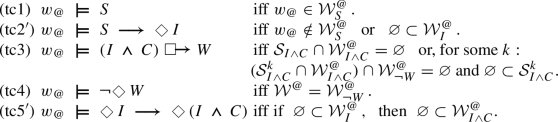(3.2)

From (tc1) and (tc2$$'$$) we have that $$\mathcal {W}^{@}_{I}$$ is not empty, and then by (tc5$$'$$), $$\mathcal {W}^{@}_{I\wedge C}$$ is not empty either. But we can fulfill again the first disjunct of (tc3) by making all worlds in $$\mathcal {W}^{@}_{I\wedge C}$$ dissimilar from $$w_{@}$$, so that $$\mathcal {S}_{I\wedge C}=\varnothing$$. We can further choose $$\mathcal {W}^{@}$$ such that W is false for every accessible world (tc4). In this arrangement every premise of pentad (3.1) is true, which shows its consistency.

To make the pentad (3.1) inconsistent, we only need to add premise 7 of (2.6).

Proof of Inconsistency. From 1 and $$2'$$ we obtain, which yieldsby virtue of $$5'$$. The conjunction with 3 yields the antecedent of 7, and again by modus ponens we deduce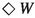, which contradicts 4. Q.e.d.

## 4 Häggqvist’s Counterfactual Refuter

Häggqvist (2009, p. 63) presents a courser modal-logical structure of destructive thought experiments, which has the three premises 5, 8 and 9 below (replacing his T with our S). Häggqvist deduces from these premises. For convenience, we add S as a fourth premise to obtain an inconsistent tetrad, which is logically equivalent to Häggqvist’s argument, and ready for comparison to Sorensen’s schemata. Here comes the Counterfactual Refuter:(4.1)

Häggqvist (ibid.) points out that “on e.g. a Lewisian semantics for counterfactuals”, premises 5 and 9 jointly imply a refutation of the counterfactual”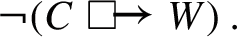(4.2)

Then by modus tollendo tollens from premise 8 and consequence (4.2) we arrive at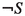, which contradicts premise 1 (S).

Thus Häggqvist’s claim is that the following is a theorem of modal logic: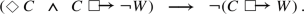(4.3)

Proof of Inconsistency. Premises 5 (S) and 9 of (4.1) yield the antecedent of proposition (4.3). We then deduce the consequent,, which is the negation of the consequent of the material conditional of premise 8. Hence by modus tollendo tollens we obtain from premise 8, as Häggqvist wanted to conclude. Of coursecontradicts premise 1 of (4.1). Q.e.d.

How to justify proposition 10? Again, the case of vacuously true counterfactuals shows that it is not a logical truth. For proposition 10 is an instance of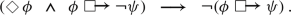(4.4)

Then suppose $$\phi$$ is true in some worlds accessible for $$w_{@}$$ yet dissimilar to $$w_{@}$$, makingtrue in $$w_{@}$$. These worlds then are not in any similarity set $$S^{k}_{\phi }$$, and via the first disjunct (i) of the truth-condition of the counterfactual (2.3), the counterfactualis rendered true. The counterfactual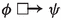is also rendered true, because the consequent no longer matters. But then the negation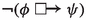is false. In this arrangement, the antecedent of (4.4) is true and the consequent false, which makes the material conditional (4.4) false. Hence proposition (4.4) is not a logical truth but a substantive premise that needs to be acknowledged.

## 5 Conclusions

We have argued for the deductive failure of all three well-known inconsistency arguments intended to capture the logical argument that accompanies destructive thought experiments: Sorensen’s two pentads (2.1) and (3.1), and Häggqvist’s tetrad (4.3). In all three cases, the reason for the failure was overlooking the vacuous truth-condition of Lewis’ standard semantics of counterfactuals. This is the critical part of the current paper. The constructive part is that we have demonstrated that premises can be added in order to yield a valid modal-logical proof of inconsistency.

Destructive thought experiments are supposed to destroy some theory, hypothesis or principle: this is a premise in Sorensen’s extended sextad and Häggqvist’s extended pentad (‘Source’ S). In order to draw this destructive conclusion, all other premises need to be accepted. One can avoid the destructive conclusion by rejecting at least one of the other premises. Sorensen has argued that each rejection of a premise of his pentad corresponds to a response that have been mounted in the literature on thought experiments.

The new premises that have surfaced in this paper are proposition 7 of (2.6) in the case of Sorensen, and proposition (4.4) in case of Häggqvist: they open new possibilities for rejecting destructive conclusions:Since the antecedent of proposition 7 of (2.6) must be accepted when the other premises of Sorensen’s pentads are accepted, its rejection amounts to claiming that this antecedent is insufficient for accepting the consequent (). When the subjunctive conditional in the antecedent is vacuously true, the consequent is indeed not guaranteed: there are then no similar $$(I\wedge C)$$-worlds; the consequent (W) then does not matter anymore, and can even be impossible. Thus, one cannot conclude that the consequent W is possible.

For proposition (4.4), which we added to Häggqvist’s Counterfactual Refuter, something similar holds. If there are only dissimilar but accessible C-worlds, the antecedent of (4.4) is true. Since then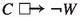is vacuously true, so is, and hence the negation of the last-mentioned counterfactual is false. We then have a material conditional with a true antecedent and false consequent, which makes this material conditional false. In both Sorensen’s cases and Häggqvist’s case, the rejection of 7 of (2.6) and (4.4) seems a natural way to make an alliance with those who deny that W is a possibility sufficiently similar to the actual world.

To illustrate this again with the Chinese room Argument (Section 2), Damper (2006), using both of Sorensen’s Refuters, meticulously categorised the responses to this thought experiment as they are found in the literature. A full summary and analysis will bear too far for current purposes of the paper; suffice it to say that our added premise 7 will add to this categorisation the logical option to reject the Chinese Room Argument on grounds of modal vacuity. That is, claiming that if it is possible that Searle hand-implements the algorithm in an indistinguishable way, and were he to do this would amount to him understanding Chinese (i.e., the antecedent (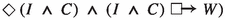), then this would not be sufficient to conclude that it is possible for him to understand Chinese. This can be defended by denying that ‘were he to do this would amount to him understanding Chinese’ is vacuously true. That is, the worlds in which Searle understands Chinese as a consequence of the antecedent are not sufficiently similar even though they are accessible.

There remains the investigation into how philosophically relevant our logic-chopping is. The premises that need to be added to make the schemas inconsistent seem plausible enough to us: we have discussed in some length what it would mean to reject them, but we cannot imagine anyone bolstering such a position. Yet promoting our lack of imagination to a philosophical impossibility sends shivers down our spine.

There is another, rather blunt way to trash our analysis that we ought to point to: leave the logical paradise that Lewis has created for us. Specifically, by removing the vacuity condition (i) from the disjunctive truth-condition for the counterfactual (2.3), and leaving condition (ii) standing as the only truth-condition. For it is the vacuity condition that we used by showing that Sorensen’s and Häqqgvist’s schemata are not inconsistent. Lewis (1973, p. 25) has discussed this stronger truth-condition but prefered truth-condition (2.3). Recently Williamson (2017) has defended the truth of counterfactuals with impossible antecedents, which thus meet the vacuity condition.

While we’re at it, we also want to point out that the status of the modal proposition of which proposition 7 of (2.6) is an instance, has recently been discussed and disputed, notably by Williamson (2017), Berto (2017), and French et al. (2022). That proposition 7 turns out to be crucial for the validity of modal-logical arguments of destructive thought experiments is a point that may not have occurred to these discussants.

In conclusion, the rejection of the propositions we added to obtain a valid inconsistency proof opens up a new logical avenue to criticise destructive thought experiments. We leave this avenue open for future inquiry: in principle the applications of the revised schemas are as numerous as there are thought experiments.